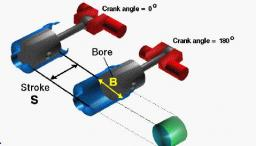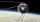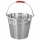# Total displacement

Calculate total displacement of the 4-cylinder engine with the diameter of the piston bore B = 6.6 cm and stroke S=2.4 cm of the piston.

Help: the crankshaft makes one revolution while the piston moves from the top of the cylinder to the bottom and back to the top. Since the piston is connected to the crankshaft, we can note the movement of the piston by the angle of rotation of the crankshaft.

Zero degrees occurs when the piston is at the top of the cylinder. Since there are 360 degrees in one revolution, the piston is at the bottom when the crank angle is 180 degrees. The distance traveled by the piston from zero degrees to 180 degrees is called the stroke - S of the piston. The piston makes four strokes and the crankshaft makes two revolutions between combustion firings. The diameter of the piston, and the inside diameter of the cylinder, is called the bore - B.

Correct result:

V =  328.4 cm3

#### Solution:We would be pleased if you find an error in the word problem, spelling mistakes, or inaccuracies and send it to us. Thank you!Tips to related online calculators
Tip: Our volume units converter will help you with the conversion of volume units.

## Next similar math problems:

• University bubbleYou'll notice that the college up slowly every other high school. In Slovakia/Czech republic a lot of people studying political science, mass media communication, social work, many sorts of management MBA. Calculate how many times more earns clever 25-yea
• Engineer KažimírThe difference between politicians-demagogues and reasonable person with at least primary education beautifully illustrated by the TV show example. "Engineer" Kažimír says that during their tenure there was a large decline in the price of natural gas, pri
• A cylinderA cylinder 108 cm high has a circumference of 24 cm. A string makes exactly 6 complete turns around the cylinder while its two ends touch the cylinder's top and bottom. (forming a spiral around the cylinder). How long is the string in cm?
• RWYCalculate the opposite direction of the runway 13. Runways are named by a number between 01 and 36, which is generally one tenth of the azimuth of the runway's heading in degrees: a runway numbered 09 points east (90°), runway 18 is south (180°), runway 2
• STRESSED wordEach letter in STRESSED is printed on identical cards, one letter per card and assembled in random order. Calculate the probability that the cards spell DESSERTS when assembled.
• The spacecraftThe spacecraft spotted a radar device at altitude angle alpha = 34 degrees 37 minutes and had a distance of u = 615km from Earth's observation point. Calculate the distance d of the spacecraft from Earth at the moment of observation. Earth is considered a
• Chickens and rabbitsIn the yard were chickens and rabbits. Together they had 27 heads and 86 legs. How many chickens and how many rabbits were in the yard?
• NonagonCalculate the area and perimeter of a regular nonagon if its radius of inscribed circle is r = 10cm
• Speed of Slovakian trainsRudolf decided to take the train from the station 'Ostratice' to 'Horné Ozorovce'. In the train timetables found train Os 5409 : km 0 Chynorany 15:17 5 Ostratice 15:23 15:23 8 Rybany 15:27 15:27 10 Dolné Naštice 15:31 15:31 14 Bánovce nad Bebravou 15:35 1Calculate the surface area and volume of a regular quadrangular pyramid: sides of bases (bottom, top): a1 = 18 cm, a2 = 6cm angle α = 60 ° (Angle α is the angle between the side wall and the plane of the base.) S =? , V =?
• Two parallel chordsIn a circle 70 cm in diameter, two parallel chords are drawn so that the center of the circle lies between the chords. Calculate the distance of these chords if one of them is 42 cm long and the second 56 cm.
• Four prismsQuestion No. 1: The prism has the dimensions a = 2.5 cm, b = 100 mm, c = 12 cm. What is its volume? a) 3000 cm2 b) 300 cm2 c) 3000 cm3 d) 300 cm3 Question No.2: The base of the prism is a rhombus with a side length of 30 cm and a height of 27 cm. The heig
• Copper windingCalculate the current flowing through the copper winding at an operating temperature of 70°C. Celsius, if the winding diameter is 1.128 mm and the winding length is 40 m. The winding is connected to 8V.
• Chained winchWhen digging a well, a chained winch is used to balance the material. An empty shackle weighs 8 kg, a full 64 kg. What force must the worker exert on the crank handle when pulling the shackle with a uniform movement from a depth of 5 m, if the shaft diame
• Distance between two citiesCar went from A to B 4h. On the way back, the car was up 15km / h faster. The return trip took 48 minutes. Shorter than the way there. Find the distance of the cities A and B.
• Z9–I–4 MO 2017Numbers 1, 2, 3, 4, 5, 6, 7, 8 and 9 were prepared for a train journey with three wagons. They wanted to sit out so that three numbers were seated in each carriage and the largest of each of the three was equal to the sum of the remaining two. The conduct
• PebbleThe aquarium with internal dimensions of the bottom 40 cm × 35 cm and a height of 30 cm is filled with two-thirds of water. Calculate how many millimeters the water level in the aquarium rises by dipping a pebble-shaped sphere with a diameter of 18 cm.